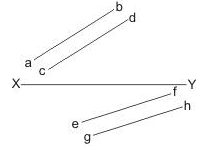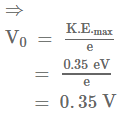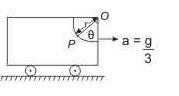Courses

# JEE(MAIN) Physics Mock Test

## 30 Questions MCQ Test JEE Main Mock Test Series 2020 & Previous Year Papers | JEE(MAIN) Physics Mock Test

Description
This mock test of JEE(MAIN) Physics Mock Test for JEE helps you for every JEE entrance exam. This contains 30 Multiple Choice Questions for JEE JEE(MAIN) Physics Mock Test (mcq) to study with solutions a complete question bank. The solved questions answers in this JEE(MAIN) Physics Mock Test quiz give you a good mix of easy questions and tough questions. JEE students definitely take this JEE(MAIN) Physics Mock Test exercise for a better result in the exam. You can find other JEE(MAIN) Physics Mock Test extra questions, long questions & short questions for JEE on EduRev as well by searching above.
QUESTION: 1

Solution:
QUESTION: 2

Solution:
QUESTION: 3

### In a transformer, the number of turns in primary coil and secondary coil are 5 and 4 respectively. If 240 V is applied on the primary coil, then the ratio of current in primary and secondary coil is

Solution:

We know that

Ns / Np =  Is / Ip , where p represents primary and s ,secondary coil

Ip / Is = 4 : 5

QUESTION: 4
The wavelength 'λ' for a particle with momentum p
Solution:
QUESTION: 5
A straight wire of length l and electric dipole moment p is bent to form a semicircle. The new dipole moment would be
Solution:
QUESTION: 6
Two charged conducting spheres of radii a and b are connected to each other by a wire. The ratio of electric fields at the surface of two spheres is
Solution:
QUESTION: 7
The net charge on a current carrying conductor is
Solution:
QUESTION: 8
Two pieces of a glass plate, placed one upon the other with a little water in between them, can not be separated easily, because of
Solution:
QUESTION: 9
A ball of mass 0.25 kg attached to the end of a string of length 1.96 m is moving in a horizontal circle. The string will break if the tension is more than 25 N. What is the maximum speed with which the ball can be moved
Solution:
QUESTION: 10

A bullet of mass m and velocity V is fired into a block of mass M and sticks to it. The final velocity would be

Solution:
QUESTION: 11
A logic gate is an electronic circuit which
Solution:
QUESTION: 12

If a person with a spring balance and a body hanging from it goes up and up in an aeroplane, then reading of weight of body as indicated by spring balance will

Solution:

Spring balance measures the weight of the body which depends upon the value of g. The height obtained by an aeroplane will not bring significant change in g so the spring balance is to read the same

QUESTION: 13
In the following question, a Statement of Assertion (A) is given followed by a corresponding Reason (R) just below it. Read the Statements carefully and mark the correct answer-
Assertion(A):A wire has a resistance of 12Ω. It is bent in the form of a circle. The effective resistance between two points on any diameter is 6Ω.
Reason(R): Equivalent resistance between two points on any diameter is in series.
Solution:
QUESTION: 14
In radioactive decay process, the negatively charged emitted $\beta$ -particles are
Solution:
QUESTION: 15
In the following question, a Statement of Assertion (A) is given followed by a corresponding Reason (R) just below it. Read the Statements carefully and mark the correct answer-
Assertion(A): Refractive index of a material depends on the frequency of the light
Reason(R): Incoming e.m. wave applies an electric field and polarises it, contributing to (∈) permittivity of the medium, depending on its frequency.
Solution:
QUESTION: 16
Blowing air with open mouth is an example of
Solution:
QUESTION: 17
A spherical ball rolls on a table without slipping. Then the fraction of its total energy associated with rotation is
Solution:
QUESTION: 18
A stationary point source of sound emits sound uniformly in all directions in a non-absorbing medium. Two points P and Q are at a distance of 4m and 9m respectively from the source. The ratio of amplitudes of the waves at P and Q is
Solution:
QUESTION: 19
Which of the following is a dimensionless quantity?
Solution:
QUESTION: 20

We have two radioactive nuclei A and B. Both convert into a stable nucleus C. Nucleus A converts into Cafter emitting two αα-particles and three β-particles. Nucleus B converts into C after emitting one αα-particle and five β-particles. At time t=0, nuclei of A are 4N0 and that of B are N0. Half life of A (into the conversion of C) is 1min and that of B is 2min. Initially number of nuclei of C are zero.

Q. What are number of nuclei of C, when number of nuclei of A and B are equal?

Solution:
QUESTION: 21

We have two radioactive nuclei A and B. Both convert into a stable nucleus C. Nucleus A converts into Cafter emitting two αα-particles and three β-particles. Nucleus B converts into C after emitting one αα-particle and five β-particles. At time t=0, nuclei of A are 4N0 and that of B are N0. Half life of A (into the conversion of C) is 1min and that of B is 2min. Initially number of nuclei of C are zero.

Q. If atomic numbers and mass numbers of A and B are Z1Z2A1 and A2 respectively. Then

Solution:
QUESTION: 22

The figure shows a surface XY separating two transparent media, medium - 1 and medium - 2. The lines aband cd represent wavefronts of a light wave travelling in medium - 1 and incident on XY. The lines ef and gh represent wavefronts of the light wave in medium - 2 after refraction.Q. Light travels as a

Solution:

The wavefronts in medium 1 are parallel to each other. Hence incident beam consists of parallel light rays. Same is true for refracted beam in the medium 2. Thus, A is correct.

QUESTION: 23

The figure shows a surface XY separating two transparent media, medium - 1 and medium - 2. The lines aband cd represent wavefronts of a light wave travelling in medium - 1 and incident on XY. The lines ef and gh represent wavefronts of the light wave in medium - 2 after refraction.Q. The phases of the light waves at cde and f are φc, φd, φeand φf respectively.
It is given that φc ≠ φf ≠ φf

Solution:
QUESTION: 24

The figure shows a surface XY separating two transparent media, medium - 1 and medium - 2. The lines aband cd represent wavefronts of a light wave travelling in medium - 1 and incident on XY. The lines ef and gh represent wavefronts of the light wave in medium - 2 after refraction.Q. Speed of light is

Solution:
QUESTION: 25

Three capacitors each of capacitance C and of breakdown voltage V are joined in series. The capacitance and breakdown voltage of the combination will be:

Solution:
QUESTION: 26

The work function of caesium metal is 2.14 eV. When light of frequency 6 x 1014Hz is incident on the metal surface, photoemission of electrons occurs. What is the stopping potential?

Solution:

Work function of caesium metal = 2.14 eV
frequency of light wave = 6 x 1014Hz

The stopping potential can be calculated as,

eV0 = K.E.maxQUESTION: 27

A body at point P is free to slide with negligible friction on the circular guide mounted in the vertical frame as shown in figure. If the frame starts from rest with a constant acceleration. The velocity of body as a function of θ isSolution:
QUESTION: 28

Drift velocity is equal to

Solution:
QUESTION: 29

For transferring a charge +Q coulomb from point A to point B separated by a distance d, the work required to be done by an external agency is -W joule. If the potential of A is V volt, the potential of B will be (in volt)

Solution:
QUESTION: 30

Two ions having equal masses but charges in the ratio 1:2 are projected perpendicular to a uniform magnetic field with speeds in the ratio 2:3. The ratio of the radii of the circular paths along which the two ions move is

Solution:

R = mv / Bq

R1 / R2 = (v1 / v2) (q2 / q1)

= 2/3 : 2/1

= 4/3